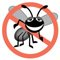# Stack Class of Package java.util

In Chapter 17, Data Structures, we learned how to build fundamental data structures, including linked lists, stacks, queues and trees. In a world of software reuse, rather than building data structures as we need them, we can often take advantage of existing data structures. In this section, we investigate class Stack in the Java utilities package (java.util).

Section 19.5.3 discussed class Vector, which implements a dynamically resizable array. Class Stack extends class Vector to implement a stack data structure. Autoboxing occurs when you add a primitive type to a Stack, because class Stack stores only references to objects. Figure 19.16 demonstrates several Stack methods. For the details of class Stack, visit java.sun.com/j2se/5.0/docs/api/java/util/Stack.html.

Figure 19.16. Stack class of package java.util.

(This item is displayed on pages 936 - 937 in the print version)

``` 1 // Fig. 19.16: StackTest.java
2 // Program to test java.util.Stack.
3 import java.util.Stack;
4 import java.util.EmptyStackException;
5
6 public class StackTest
7 {
8 public StackTest()
9 {
10 Stack< Number > stack = new Stack< Number >();
11
12 // create numbers to store in the stack
13 Long longNumber = 12L;
14 Integer intNumber = 34567;
15 Float floatNumber = 1.0F;
16 Double doubleNumber = 1234.5678;
17
18 // use push method
19 stack.push( longNumber ); // push a long
20 printStack( stack );
21 stack.push( intNumber ); // push an int
22 printStack( stack );
23 stack.push( floatNumber ); // push a float
24 printStack( stack );
25 stack.push( doubleNumber ); // push a double
26 printStack( stack );
27
28 // remove items from stack
29 try
30 {
31 Number removedObject = null;
32
33 // pop elements from stack
34 while ( true )
35 {
36 removedObject = stack.pop(); // use pop method
37 System.out.printf( "%s popped
", removedObject );
38 printStack( stack );
39 } // end while
40 } // end try
41 catch ( EmptyStackException emptyStackException )
42 {
43 emptyStackException.printStackTrace();
44 } // end catch
45 } // end StackTest constructor
46
47 private void printStack( Stack< Number > stack )
48 {
49 if ( stack.isEmpty() )
50 System.out.print( "stack is empty

" ); // the stack is empty
51 else // stack is not empty
52 {
53 System.out.print( "stack contains: " );
54
55 // iterate through the elements
56 for ( Number number : stack )
57 System.out.printf( "%s ", number );
58
59 System.out.print( "(top)

" ); // indicates top of the stack
60 } // end else
61 } // end method printStack
62
63 public static void main( String args[] )
64 {
65 new StackTest();
66 } // end main
67 } // end class StackTest
```

 ```stack contains: 12 (top) stack contains: 12 34567 (top) stack contains: 12 34567 1.0 (top) stack contains: 12 34567 1.0 1234.5678 (top) 1234.5678 popped stack contains: 12 34567 1.0 (top) 1.0 popped stack contains: 12 34567 (top) 34567 popped stack contains: 12 (top) 12 popped stack is empty java.util.EmptyStackException at java.util.Stack.peek(Unknown Source) at java.util.Stack.pop(Unknown Source) at StackTest.(StackTest.java:36) at StackTest.main(StackTest.java:65) ```

Line 10 of the constructor creates an empty Stack of type Number. Class Number (in package java.lang) is the superclass of most wrapper classes (e.g., Integer, Double) for the primitive types. By creating a Stack of Number, objects of any class that extends the Number class can be pushed onto the stack. Lines 19, 21, 23 and 25 each call Stack method push to add objects to the top of the stack. Note the literals 12L (line 13) and 1.0F (line 15). Any integer literal that has the suffix L is a long value. An integer literal without a suffix is an int value. Similarly, any floating-point literal that has the suffix F is a float value. A floating-point literal without a suffix is a double value. You can learn more about numeric literals in the Java Language Specification at java.sun.com/docs/books/jls/second_edition/html/expressions.doc.html#224125.

An infinite loop (lines 3439) calls Stack method pop to remove the top element of the stack. The method returns a Number reference to the removed element. If there are no elements in the Stack, method pop throws an EmptyStackException, which terminates the loop. Class Stack also declares method peek. This method returns the top element of the stack without popping the element off the stack.

Line 49 calls Stack method isEmpty (inherited by Stack from class Vector) to determine whether the stack is empty. If it is empty, the method returns true; otherwise, the method returns false.

Method printStack (lines 4761) uses the enhanced for statement to iterate through the elements in the stack. The current top of the stack (the last value pushed onto the stack) is the first value printed. Because class Stack extends class Vector, the entire public interface of class Vector is available to clients of class Stack.

Error-Prevention Tip 19.1Because Stack extends Vector, all public Vector methods can be called on Stack objects, even if the methods do not represent conventional stack operations. For example, Vector method add can be used to insert an element anywhere in a stackan operation that could "corrupt" the stack. When manipulating a Stack, only methods push and pop should be used to add elements to and remove elements from the Stack, respectively.Java How to Program (6th Edition) (How to Program (Deitel))
ISBN: 0131483986
EAN: 2147483647
Year: 2003
Pages: 615